# Step-up, Step-down, and Isolation Transformers

## AC Electric Circuits

• #### Question 1

Calculate the voltage output by the secondary winding of a transformer if the primary voltage is 35 volts, the secondary winding has 4500 turns, and the primary winding has 355 turns.

Vsecondary =

• #### Question 2• #### Question 3

Calculate the number of turns needed in the secondary winding of a transformer to transform a primary voltage of 300 volts down to a secondary voltage of 180 volts, if the primary winding has 1150 turns of wire.

Nsecondary =

• #### Question 4

Predict how all component voltages and currents in this circuit will be affected as a result of the following faults. Consider each fault independently (i.e. one at a time, no multiple faults):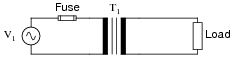Transformer T1 primary winding fails open:
Transformer T1 primary winding fails shorted:
Transformer T1 secondary winding fails open:

For each of these conditions, explain why the resulting effects will occur.

• #### Question 5

Suppose 1200 turns of copper wire are wrapped around one portion of an iron hoop, and 3000 turns of wire are wrapped around another portion of that same hoop. If the 1200-turn coil is energized with 15 volts AC (RMS), how much voltage will appear between the ends of the 3000-turn coil?

• #### Question 6

Calculate the voltage output by the secondary winding of a transformer if the primary voltage is 230 volts, the secondary winding has 290 turns, and the primary winding has 1120 turns.

Vsecondary =

• #### Question 7

Calculate the source current and load current in this transformer circuit:• #### Question 8

If a coil of insulated wire is wrapped around an iron core, an inductance will be formed. Even if the wire has negligible resistance, the current through the coil from an AC source will be limited by the inductive reactance (XL) of the coil, as the magnetic flux in the iron core oscillates back and forth to induce a counter-EMF: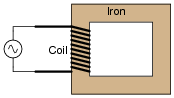Plot the instantaneous magnetic flux (φ) waveform in the iron core corresponding to the instantaneous applied voltage (v) shown in this graph: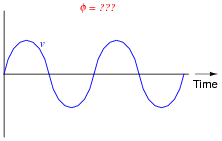• #### Question 9

If we energize an inductor’s coil with an oscillating (AC) voltage, we will generate an oscillating magnetic flux in the inductor core: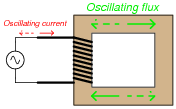If we wrap a second coil of wire around the same magnetic core as the first (inductor) coil, we set up a situation where mutual inductance exists: a change of current through one coil induces a voltage in the other, and visa-versa. This, obviously, will result in an AC voltage being induced in the second wire coil: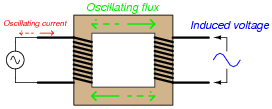What name is given to such a device, with two coils of wire sharing a common magnetic flux? Also, plot both the magnetic flux waveform and the secondary (induced) voltage waveform on the same graph as the primary (applied) voltage waveform: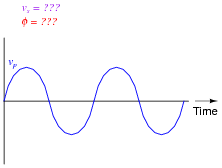• #### Question 10

Shown here is a schematic diagram of a transformer powering a resistive load, at the exact moment in time where the primary winding’s voltage is at its positive ( ) peak:Identify the polarity of voltage across the load resistor at this exact moment in time, as well as the direction of current in each of the windings.

• #### Question 11

The ignition coil of a gasoline-powered internal combustion automobile engine is an example of a transformer, although it is not powered by alternating current. Explain how a transformer may be operated on electricity that is not AC: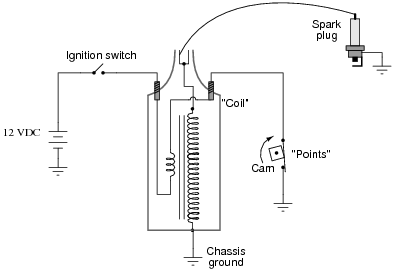• #### Question 12

Something has failed in this circuit, because the light bulb does not light up when the switch is closed: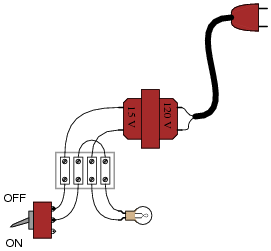What type(s) of transformer fault(s) would cause a problem like this, and how might you verify using a multimeter?

• #### Question 13

Industrial control power transformers are used to step down 480 or 240 volts to a level more acceptable for relay control circuitry: usually 120 volts. Some control power transformers are built with multiple primary windings, to facilitate connection to either a 480 volt or 240 volt AC power source:Such transformers are usually advertised as having “240 × 480” primary windings, the “×” symbol representing two independent windings with four connection points (H1 through H4).

Show the connections on the four “H” terminals necessary for 240 volt operation, and also for 480 volt operation, on the following illustrations: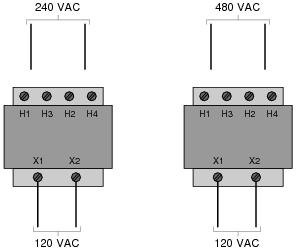• #### Question 14

If an isolation transformer (a transformer with the same number of “turns” in the primary and secondary coils) is connected between an AC source and an AC load, we will measure the same voltage and the same current at both source and load terminals:If we calculate power output by the source and power dissipated by the load, the value is the same: 420 Watts (P = IV).

Now suppose we analyze a circuit containing a step-up transformer (one with more turns of wire in the secondary coil than in the primary coil). With a step-up transformer, the load voltage will be greater than the supply voltage. In this example, I show a step-up transformer with a 1:2 step ratio:Assuming the load resistance is completely different from the first (isolation transformer) circuit, what can you deduce about the load current and the power (both source and load) in this circuit? Is the load current less than the source current? Is the load current greater than the source current? Is the load power greater than the source power? Explain your answers.

• #### Question 15

Calculate all listed values for this transformer circuit:Vprimary =
Vsecondary =
Iprimary =
Isecondary =

Explain whether this is a step-up, step-down, or isolation transformer, and also explain what distinguishes the “primary” winding from the “secondary” winding in any transformer.

• #### Question 16

Calculate all listed values for this transformer circuit: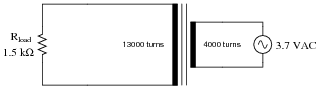Vprimary =
Vsecondary =
Iprimary =
Isecondary =

Explain whether this is a step-up, step-down, or isolation transformer, and also explain what distinguishes the “primary” winding from the “secondary” winding in any transformer.

• #### Question 17

In a typical step-up or step-down transformer, the higher-voltage winding usually uses finer gauge wire than the lower-voltage winding. Explain why this is.

• #### Question 18

A mechanic goes to school and takes a course in AC electric circuits. Upon learning about step-up and step-down transformers, he makes the remark that “Transformers act like electrical versions of gears, with different ratios.”

What does the mechanic mean by this statement? What exactly is a “gear ratio,” and is this an accurate analogy for a transformer?

• #### Question 19

Explain how this special transformer is able to control power to the light bulb:What advantages might there be to using a transformer to control AC power, as opposed to a variable resistor?

Note: a similar type of device is called a Variac, and it enjoys the same advantages of AC power control as the variable transformer shown in the question.

• #### Question 20

In this variable-voltage transformer circuit, the input voltage (120 VAC) is switched to different “taps” on the transformer’s primary winding to create different step-down ratios.While it is possible to “tap” the secondary winding of the transformer to achieve different output voltages instead of the primary, there is a good reason for locating the switch in the primary side of the circuit. Identify this practical reason.

• #### Question 21

Suppose a power system were delivering AC power to a resistive load drawing 150 amps:Calculate the load voltage, load power dissipation, the power dissipated by the wire resistance (Rwire), and the overall power efficiency (η = Pload/Psource).

Plines =
η =

Now, suppose we were to use a pair of perfectly efficient 10:1 transformers to step the voltage up for transmission, and back down again for use at the load. Re-calculate the load voltage, load power, wasted power, and overall efficiency of this system:Plines =
η =
• #### Question 22

Shunt resistors are commonly used to measure current in power circuits, by producing a small voltage drop in direct proportion to the circuit current. They are especially useful for measuring complex current waveforms in AC circuits, since they do not distort the waveform at all.

Suppose you wished to measure the current waveform in this power circuit by using an oscilloscope to measure voltage dropped across the shunt resistor: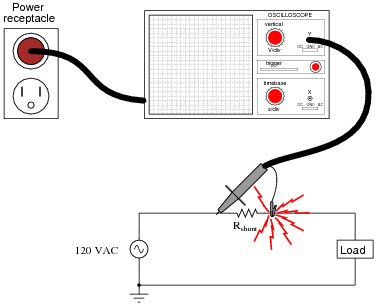If you connect an oscilloscope to the power circuit as shown, very bad things will happen, like the oscilloscope’s ground clip melting, with lots of sparks!

After replacing the damaged probe assembly, and taking a long break to soothe your nerves, an experienced technician suggests you plug the oscilloscope’s power cord into an isolation transformer to avoid this problem in the future. Explain what an isolation transformer is, why it prevents the short-circuit problem experienced in this circuit, and what precautions must be taken when using one.

• #### Question 23

Suppose you need to power a 120 volt, 600 watt heating element from a 240 volt source. You happen to have several 240V/120V step-down transformers on hand, but each one is only rated at 400 VA. Draw a schematic diagram showing how multiple transformers could be used to match the 120 volt load to the 240 volt source.

• #### Question 24

Explain how the construction of a step-down transformer differs from that of a step-up transformer.

• #### Question 25

Explain how the construction of an isolation transformer differs from that of a step-up or step-down transformer.

• #### Question 26

When calculating power in transformer circuits, how do the primary and secondary circuit powers (Pprimary = VprimaryIprimary and Psecondary = VsecondaryIsecondary) compare with each other? Is one greater than the other? If so, which one, and why?

• #### Question 27

Explain why transformers are used extensively in long-distance power distribution systems. What advantage do they lend to a power system?

• #### Question 28

Are the transformers connecting power plant generators to high-voltage power lines considered step-up or step-down? Explain your answer.

• #### Question 29

A soldering gun is a tool used to quickly heat electrical connections for soldering. Too bulky for printed-circuit board (PCB) applications, it is better suited for point-to-point wiring applications where large wires are to be joined to metal lugs and to other wires.

In addition to being a useful soldering tool, this device is also an excellent example of a step-down transformer. Explain how the construction of a soldering gun employs a step-down transformer (with a very large step ratio!) to generate high temperatures at the soldering tip.

• #### Question 30

The circuit shown here has a problem: the lamp does not light up, even though the AC power source is known to be good. You know that the circuit used to work just fine, so it is designed properly. Something in it has failed:Identify one component or wire fault that could account for the lamp not lighting, and describe how you would use test equipment to verify that fault.

One failed wire or component in the circuit that could possibly account for the problem, and the type of fault (open or short) you suspect it would be.
Identify the type of test measurement you would take on this circuit, and where you would take it (identify the test points you would measure between) to verify the suspected fault.
• #### Question 31

Suppose a step-down transformer fails due to an accidental short-circuit on the secondary (load) side of the circuit:That the transformer actually failed as a result of the short is without any doubt: smoke was seen coming from it, shortly before current in the circuit stopped. A technician removes the burned-up transformer and does a quick continuity check of both windings to verify that it has failed open. What she finds is that the primary winding is open but that the secondary winding is still continuous. Puzzled at this finding, she asks you to explain how the primary winding could have failed open while the secondary winding is still intact, if indeed the short occurred on the secondary side of the circuit. What would you say? How is it possible that a fault on one side of the transformer caused the other side to be damaged?

• #### Question 32

An AC motor receives reduced voltage through a step-down transformer so it may properly operate on a 277 volt source:After years of trouble-free operation, something fails. Now, the motor refuses to operate when both switches are closed. A technician takes four voltage measurements between the following test points with both switches in the “on” position:

 Step Measurement 1 VTP2−Gnd = 277 V 2 VTP3−Gnd = 277 V 3 VTP5−Gnd = 0 V 4 VTP4−Gnd = 0 V

Complete this expanded table, following the technician’s steps in the same order as the voltage measurements were taken, labeling each component’s status as either “O” (possibly open), “S” (possibly shorted), or “OK” (known to be good). The first row of the table should contain many possible fault labels (because with little data there are many possibilities), but as more measurements are taken you should be able to limit the possibilities. Assume that only one component is faulted.

 Step Measurement SW1 Fuse Primary Secondary SW2 Motor 1 VTP2−Gnd = 277 V 2 VTP3−Gnd = 277 V 3 VTP5−Gnd = 0 V 4 VTP4−Gnd = 0 V

• #### Question 33

Ethernet is a popular communications standard for many digital devices, personal computers included. Originally, Ethernet was intended to be a network standard for conveying digital data only, without power. In later years, however, upgrades to the standard allowed DC power to be conveyed over the same wire pairs. The IEEE standard 802.3af is one example of a power-over-Ethernet standard.

Shown here is a schematic showing how two Ethernet devices connect together over a Category 5 (“Cat 5”) twisted-pair cable, with no DC power conveyed over the cabling:Digital data consists of voltage pulses over time (AC, essentially), conducted between the two devices over two sets of twisted-pair wires.

The next schematic shows the 802.3af standard allowing both DC power and digital data to be communicated over the same pairs of wires. Note the use of transformers at each device: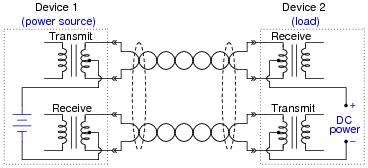Explain what function(s) the transformers provide in this system, and how they allow DC power to travel through the wire pairs from source to load without interfering with the Ethernet data signals, which are AC.

• #### Question 34

Find one or two real transformers and bring them with you to class for discussion. Identify as much information as you can about your transformers prior to discussion:

Winding ratio(s)
Winding resistances
Voltage rating of each winding
Current rating of each winding
Frequency rating
Application (power, signal, audio, etc.)
Type (iron core, air core, etc.)